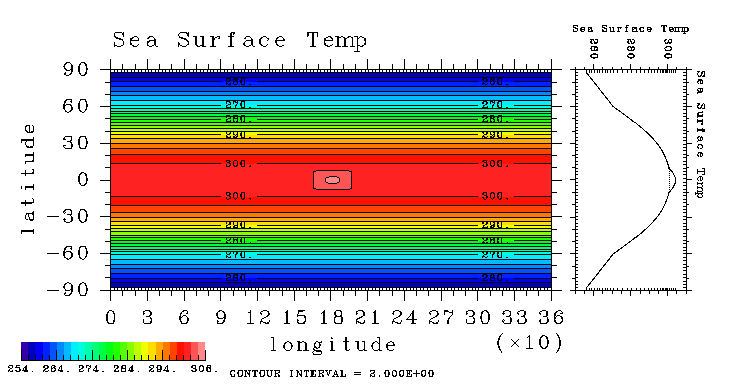Introduction Index Design of Ensemble Experiment

## Model

The numerical model utilized here is the same as that of Hosaka et al. (1998), which is AGCM5 of the GFD-DENNOU CLUB edition that is based on the three-dimensional primitive system on a sphere. The horizontal resolution is T42 (truncated at wavenumber 42), and the number of vertical levels is 16. The cumulus parameterization is convectiv adjustment (Manabe et al., 1965). The longwave absorption is represented by three bands of water vapor and one band of dry air. The absorption coefficients are empirically chosen so that the cooling profile of the atmosphere roughly resembles the observed one. Scattering of longwave radiation, scattering by clouds, and absorption/scattering of shortwave radiation are not included. The vertical mixing is represented by the level II scheme of Mellor and Yamada (1974). The surface fluxes of momentum, heat and water vapor are evaluated by the bulk formula.

The distribution of SST is fixed and given in the same way as that of Hosaka et al. (1998); A warm SST area is placed at the equator centered at 180E of a sphere covered with an SST distribution symmetric around the equator and zonally uniform (Figure 1). The shape of the warm SST area is a rectangle with the longitudinal and latitudinal extents of 40 degree and 20 degree, respectively. The value of temperature of the warm area is the highest at the center and gradually decreases toward the edge. On the actual grid point, it is 3.4K higher than the surroundings.

 Figure 1: Horizontal distribution of SST (left panel) and latitudinal profile of SST (right panel). Unit is K. Dashed line and solid line in the right panel indicate the basic profile and the profile at the centered longitude of the warm SST area, respectively.Introduction Index Design of Ensemble Experiment